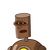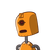# in triangle ABC , angle A = 100 degree and AB = AC . find angle B​

in triangle ABC , angle A = 100 degree and AB = AC . find angle B​

### 2 thoughts on “in triangle ABC , angle A = 100 degree and AB = AC . find angle B​”

1.Step-by-step explanation:

let angle b= x

ACQ:-

a+b+c = 180(angle sum property)

100 + x+x = 180(angle a = angle b (angles substituded by equal lines))

2x = 80

x =40

hope you like it ,please mark me brainliest

2.angle B is 40°

Step-by-step explanation:

STEP BY STEP EXPLANATION:

Angle a=100°—-(1.)

AB=AC

So, angle B=angleC —–(2.) (because angles opposite to equal sides are equal.)

Thus, by (1) and (2) we can conclude that

angle A+angleB+angleC=180°(Angle Sum Property)

so, 100+angleB+angleB=180

100+2(angle B)=180

2(angleB)=180-100

2(angle B)=80

angle B=80/2

angle B=40°

______________________☆☆

HOPE IT HELPED ^_